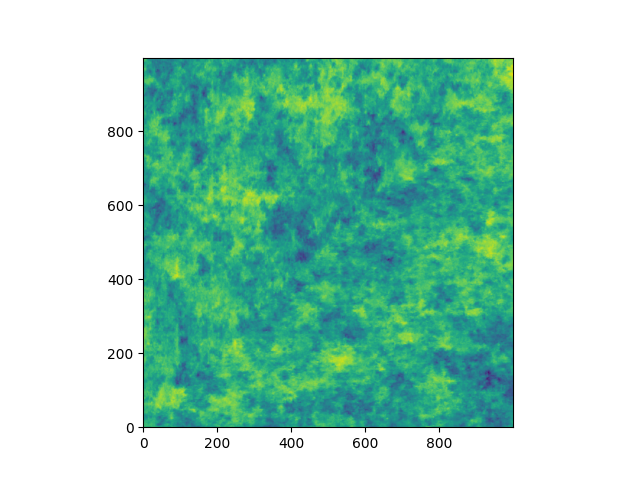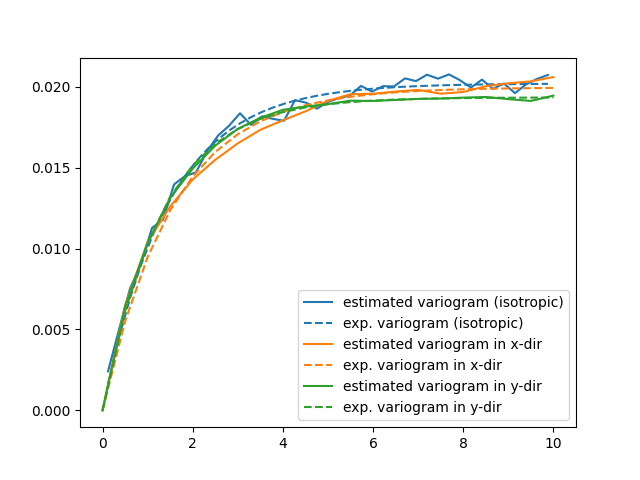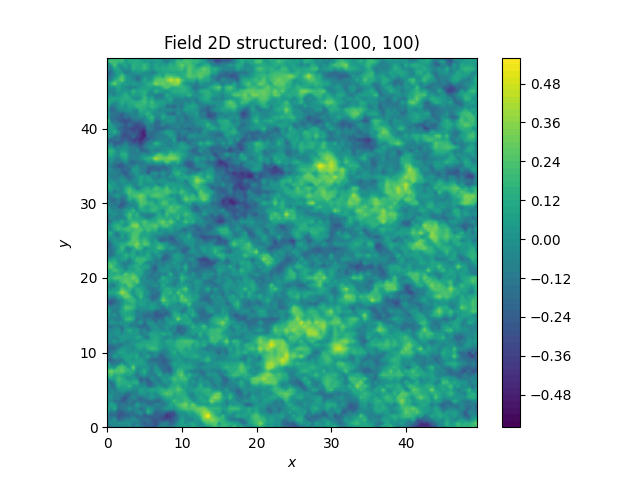# Analyzing the Herten Aquifer with GSTools

This example is going to be a bit more extensive and we are going to do some basic data preprocessing for the actual variogram estimation. But this example will be self-contained and all data gathering and processing will be done in this example script.

## The Data

We are going to analyse the Herten aquifer, which is situated in Southern Germany. Multiple outcrop faces where surveyed and interpolated to a 3D dataset. In these publications, you can find more information about the data:

Bayer, Peter; Comunian, Alessandro; Höyng, Dominik; Mariethoz, Gregoire (2015): Physicochemical properties and 3D geostatistical simulations of the Herten and the Descalvado aquifer analogs. PANGAEA, https://doi.org/10.1594/PANGAEA.844167,
Supplement to: Bayer, P et al. (2015): Three-dimensional multi-facies realizations of sedimentary reservoir and aquifer analogs. Scientific Data, 2, 150033, https://doi.org/10.1038/sdata.2015.33

## Retrieving the Data

To begin with, we need to download and extract the data. Therefore, we are going to use some built-in Python libraries. For simplicity, many values and strings will be hardcoded.

You don’t have to execute the `download_herten` and `generate_transmissivity` functions, since the only produce the `herten_transmissivity.gz` and `grid_dim_origin_spacing.txt`, which are already present.

```import os

import matplotlib.pyplot as plt
import numpy as np

import gstools as gs

VTK_PATH = os.path.join("Herten-analog", "sim-big_1000x1000x140", "sim.vtk")
```
```def download_herten():
import urllib.request
import zipfile

data_filename = "data.zip"
data_url = (
"http://store.pangaea.de/Publications/"
"Bayer_et_al_2015/Herten-analog.zip"
)
urllib.request.urlretrieve(data_url, "data.zip")
# extract the "big" simulation
with zipfile.ZipFile(data_filename, "r") as zf:
zf.extract(VTK_PATH)
```
```def generate_transmissivity():
"""Generate a file with a transmissivity field from the HERTEN data."""
import shutil

import pyvista as pv

herten = mesh.point_data["facies"].reshape(mesh.dimensions, order="F")
# conductivity values per fazies from the supplementary data
cond = 1e-4 * np.array(
[2.5, 2.3, 0.61, 260, 1300, 950, 0.43, 0.006, 23, 1.4]
)
# asign the conductivities to the facies
herten_cond = cond[herten]
# Next, we are going to calculate the transmissivity,
# by integrating over the vertical axis
herten_trans = np.sum(herten_cond, axis=2) * mesh.spacing
# saving some grid informations
grid = [mesh.dimensions[:2], mesh.origin[:2], mesh.spacing[:2]]
print("Saving the transmissivity field and grid information")
np.savetxt("herten_transmissivity.gz", herten_trans)
np.savetxt("grid_dim_origin_spacing.txt", grid)
# Some cleanup. You can comment out these lines to keep the downloaded data
os.remove("data.zip")
shutil.rmtree("Herten-analog")
```

You can uncomment the following two calls, so the data is downloaded and processed again.

```# download_herten()
# generate_transmissivity()
```

## Analyzing the data

The Herten data provides information about the grid, which was already used in the previous code block. From this information, we can create our own grid on which we can estimate the variogram. As a first step, we are going to estimate an isotropic variogram, meaning that we will take point pairs from all directions into account. An unstructured grid is a natural choice for this. Therefore, we are going to create an unstructured grid from the given, structured one. For this, we are going to write another small function

```herten_log_trans = np.log(np.loadtxt("herten_transmissivity.gz"))
dim, origin, spacing = np.loadtxt("grid_dim_origin_spacing.txt")

# create a structured grid on which the data is defined
x_s = np.arange(origin, origin + dim * spacing, spacing)
y_s = np.arange(origin, origin + dim * spacing, spacing)
# create the corresponding unstructured grid for the variogram estimation
x_u, y_u = np.meshgrid(x_s, y_s)
```

Let’s have a look at the transmissivity field of the Herten aquifer

```plt.imshow(herten_log_trans.T, origin="lower", aspect="equal")
plt.show()
```## Estimating the Variogram

Finally, everything is ready for the variogram estimation. For the unstructured method, we have to define the bins on which the variogram will be estimated. Through expert knowledge (i.e. fiddling around), we assume that the main features of the variogram will be below 10 metres distance. And because the data has a high spatial resolution, the resolution of the bins can also be high. The transmissivity data is still defined on a structured grid, but we can simply flatten it with `numpy.ndarray.flatten`, in order to bring it into the right shape. It might be more memory efficient to use `herten_log_trans.reshape(-1)`, but for better readability, we will stick to `numpy.ndarray.flatten`. Taking all data points into account would take a very long time (expert knowledge *wink*), thus we will only take 2000 datapoints into account, which are sampled randomly. In order to make the exact results reproducible, we can also set a seed.

```bins = gs.standard_bins(pos=(x_u, y_u), max_dist=10)
bin_center, gamma = gs.vario_estimate(
(x_u, y_u),
herten_log_trans.reshape(-1),
bins,
sampling_size=2000,
sampling_seed=19920516,
)
```

The estimated variogram is calculated on the centre of the given bins, therefore, the `bin_center` array is also returned.

## Fitting the Variogram

Now, we can see, if the estimated variogram can be modelled by a common variogram model. Let’s try the `Exponential` model.

```# fit an exponential model
fit_model = gs.Exponential(dim=2)
fit_model.fit_variogram(bin_center, gamma, nugget=False)
```

Finally, we can visualise some results. For quickly plotting a covariance model, GSTools provides some helper functions.

```ax = fit_model.plot(x_max=max(bin_center))
ax.plot(bin_center, gamma)
```That looks like a pretty good fit! By printing the model, we can directly see the fitted parameters

```print(fit_model)
```
```Exponential(dim=2, var=0.0202, len_scale=1.45, nugget=0.0)
```

With this data, we could start generating new ensembles of the Herten aquifer with the `SRF` class.

## Estimating the Variogram in Specific Directions

Estimating a variogram on a structured grid gives us the possibility to only consider values in a specific direction. This could be a first test, to see if the data is anisotropic. In order to speed up the calculations, we are going to only use every 10th datapoint and for a comparison with the isotropic variogram calculated earlier, we only need the first 21 array items.

```# estimate the variogram on a structured grid
# use only every 10th value, otherwise calculations would take very long
x_s_skip = np.ravel(x_s)[::10]
y_s_skip = np.ravel(y_s)[::10]
herten_trans_skip = herten_log_trans[::10, ::10]
```

With this much smaller data set, we can immediately estimate the variogram in the x- and y-axis

```gamma_x = gs.vario_estimate_axis(herten_trans_skip, direction="x")
gamma_y = gs.vario_estimate_axis(herten_trans_skip, direction="y")
```

With these two estimated variograms, we can start fitting `Exponential` covariance models

```x_plot = x_s_skip[:21]
y_plot = y_s_skip[:21]
# fit an exponential model
fit_model_x = gs.Exponential(dim=2)
fit_model_x.fit_variogram(x_plot, gamma_x[:21], nugget=False)
fit_model_y = gs.Exponential(dim=2)
fit_model_y.fit_variogram(y_plot, gamma_y[:21], nugget=False)
```

Now, the isotropic variogram and the two variograms in x- and y-direction can be plotted together with their respective models, which will be plotted with dashed lines.

```plt.figure()  # new figure
(line,) = plt.plot(bin_center, gamma, label="estimated variogram (isotropic)")
plt.plot(
bin_center,
fit_model.variogram(bin_center),
color=line.get_color(),
linestyle="--",
label="exp. variogram (isotropic)",
)

(line,) = plt.plot(x_plot, gamma_x[:21], label="estimated variogram in x-dir")
plt.plot(
x_plot,
fit_model_x.variogram(x_plot),
color=line.get_color(),
linestyle="--",
label="exp. variogram in x-dir",
)

(line,) = plt.plot(y_plot, gamma_y[:21], label="estimated variogram in y-dir")
plt.plot(
y_plot,
fit_model_y.variogram(y_plot),
color=line.get_color(),
linestyle="--",
label="exp. variogram in y-dir",
)

plt.legend()
plt.show()
```The plot might be a bit cluttered, but at least it is pretty obvious that the Herten aquifer has no apparent anisotropies in its spatial structure.

```print("semivariogram model (isotropic):\n", fit_model)
print("semivariogram model (in x-dir.):\n", fit_model_x)
print("semivariogram model (in y-dir.):\n", fit_model_y)
```
```semivariogram model (isotropic):
Exponential(dim=2, var=0.0202, len_scale=1.45, nugget=0.0)
semivariogram model (in x-dir.):
Exponential(dim=2, var=0.0199, len_scale=1.55, nugget=0.0)
semivariogram model (in y-dir.):
Exponential(dim=2, var=0.0193, len_scale=1.31, nugget=0.0)
```

## Creating a Spatial Random Field from the Herten Parameters

With all the hard work done, it’s straight forward now, to generate new Herten-like realisations

```# create a spatial random field on the low-resolution grid
srf = gs.SRF(fit_model, seed=19770928)
srf.structured([x_s_skip, y_s_skip])
ax = srf.plot()
ax.set_aspect("equal")
```That’s pretty neat!

Total running time of the script: ( 0 minutes 3.835 seconds)

Gallery generated by Sphinx-Gallery